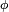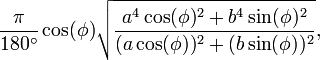# Finding Cities within ‘X’ Kilometers (or Miles)

This may or may not be clear, leave me a comment if I am off base, or you need more information. Perhaps there is a solution out there already for what I want in PHP.

I am looking for a function that will add or subtract a distance from a longitude OR latitude value.

Reason: I have a database with all Latitudes and Longitudes in it and want to form a query to extract all cities within X kilometers (or miles). My query would look something like this…

```Select * From Cities Where (Longitude > X1 and Longitude < X2) And (Latitude > Y1 and Latitude < Y2)

Where X1 = Longitude - (distance)
Where X2 = Longitude + (distance)

Where Y1 = Latitude - (distance)
Where Y2 = Latitude + (distance)```

I am working in PHP, with a MySql Database.

Open to any suggestions also! 🙂

Contents

### Method 1

This is a MySQL query that will do exactly what you want. Keep in mind things like this are approximations generally, as the earth is not perfectly spherical nor does this take into account mountains, hills, valleys, etc.. We use this code on AcademicHomes.com with PHP and MySQL, it returns records within \$radius miles of \$latitude, \$longitude.

```\$res = mysql_query("SELECT
*
FROM
your_table
WHERE
(
(69.1 * (latitude - " . \$latitude . ")) *
(69.1 * (latitude - " . \$latitude . "))
) + (
(69.1 * (longitude - " . \$longitude . ") * COS(" . \$latitude . " / 57.3)) *
(69.1 * (longitude - " . \$longitude . ") * COS(" . \$latitude . " / 57.3))
) < " . pow(\$radius, 2) . "
ORDER BY
(
(69.1 * (latitude - " . \$latitude . ")) *
(69.1 * (latitude - " . \$latitude . "))
) + (
(69.1 * (longitude - " . \$longitude . ") * COS(" . \$latitude . " / 57.3)) *
(69.1 * (longitude - " . \$longitude . ") * COS(" . \$latitude . " / 57.3))
) ASC");```

### Method 2

EDIT: If you have, somewhere, a list of all of the cities in the world along with their lat. and long. values, you can do a lookup. In this case, see my first link below for the formula to calculate the width of one longitudinal degree at latitude:Honestly, the complications behind this problem are such that you’d be far better off using a service such as Google Maps to get your data. Specifically, the Earth is not a perfect sphere, and the distance between two degrees varies as you are closer to / further from the equator.

See http://en.wikipedia.org/wiki/Geographic_coordinate_system for examples of what I mean, and check out the Google Maps API.

### Method 3

Depending on how many cities you are including, you can precompute the list. We do this here for an internal application where an inaccuracy of +100m is too much for our setup. It works by having a two key table of location1, location2, distance. We can then pull back locations x distance from location1 very quickly.

Also since the calcs can be done offline, it doesn’t impact the running of the system. Users also get faster results.

### Method 4

I Tried using the above code, and the answers were off by too much when the distance between points was in the 20-30 mile range, and I’m ok with a few miles of error. Talked with a mapping buddy of mine and we came up with this one instead. The code is python, but you can translate it pretty easily. In order to avoid the constant conversion to radians, I redid my database, converting the lat/lng points from degrees to Radians. The nice part about this is that the largest part of the math is mostly done once.

```ra = 3963.1906 # radius @ equator in miles, change to km  if you want distance in km
rb = 3949.90275  # radius @ poles in miles, change to km  if you want distance in km
ra2 = ra * ra
rb2 = rb * rb

phi = self.lat

big_ol_constant = (math.pow(ra2*math.cos(phi), 2) + pow(rb2*math.sin(phi), 2))/ (pow(ra*math.cos(phi), 2) + pow(rb*math.sin(phi), 2))

sqlWhere = "%(distance)g > sqrt((power(lat - %(lat)g,2) + power(lng-%(lng)g,2)) * %(big_ol_constant)g)" % {
'big_ol_constant': big_ol_constant, 'lat': self.lat, 'lng': self.lng, 'distance': distance}

# This is the Django portion of it, where the ORM kicks in.  sqlWhere is what you would put after the WHERE part of your SQL Query.
qs = ZipData.objects.extra(where=[sqlWhere]);```

Seems to be very accurate when distance apart is small, and within 10 miles or so as the distance grows to 200 miles, (of course by then, you have issues with “as the crow flies” vs “paved roads”).

Here is the model ZipData that I mention above.

```class ZipData(models.Model):
zipcode = ZipCodeField(null=False, blank=False, verbose_name="ZipCode", primary_key=True)
city = models.CharField(max_length=32, null=False, blank=False)
state = models.CharField(max_length=2)
lat = models.FloatField(null=False, blank=False)
lng = models.FloatField(null=False, blank=False)```

An extra note, is that you can gets LOTS of geo data related to postal codes at GeoNames.org and they even have some webservice APIs you can use as well.

### Method 5

• Calculate the distance using the mathematical formula (treat X1-X2 and Y1-Y2) as vectors.
• Create a lookup table in advance with all the combinations and keep the distances.

### Method 6

lessthandot.com actually has 3 different ways to do this. you’ll have to scroll through the blogs a little but they’re there.
http://blogs.lessthandot.com/

### Method 7

The function below is from the nerddinner‘s (ASP.NET MVC sample application available on codeplex) database (MSSQL).

```ALTER FUNCTION [dbo].[DistanceBetween] (@Lat1 as real,
@Long1 as real, @Lat2 as real, @Long2 as real)
RETURNS real
AS
BEGIN

SET @dLat1InRad = @Lat1 * (PI()/180.0);
SET @dLong1InRad = @Long1 * (PI()/180.0);
SET @dLat2InRad = @Lat2 * (PI()/180.0);
SET @dLong2InRad = @Long2 * (PI()/180.0);

DECLARE @dLongitude as float(53);
DECLARE @dLatitude as float(53);
/* Intermediate result a. */
DECLARE @a as float(53);
SET @a = SQUARE (SIN (@dLatitude / 2.0)) + COS (@dLat1InRad)
* SQUARE(SIN (@dLongitude / 2.0));
/* Intermediate result c (great circle distance in Radians). */
DECLARE @c as real;
SET @c = 2.0 * ATN2 (SQRT (@a), SQRT (1.0 - @a));
/* SET kEarthRadius = 3956.0 miles */
SET @kEarthRadius = 6376.5;        /* kms */

DECLARE @dDistance as real;
SET @dDistance = @kEarthRadius * @c;
return (@dDistance);
END```

I am guessing this could be helpful.

### Method 8

You can use Pythagoras’ Theorem to calculate the proximity of two pairs of lat/lon points.

If you have two locations (Alpha and Beta) you can calculate their distance apart with:

`SQRT( POW(Alpha_lat - Beta_lat,2) + POW(Alpha_lon - Beta_lon,2) )`

### Method 9

Using the setup from the following URL, Ive built the query below. (Please note Im using codeIgnitor to query the database)

http://howto-use-mysql-spatial-ext.blogspot.com/2007/11/using-circular-area-selection.html

```function getRadius(\$point="POINT(-29.8368 30.9096)", \$radius=2)
{
\$km = 0.009;
\$center = "GeomFromText('\$point')";
\$bbox = "CONCAT('POLYGON((',
))')";

\$query = \$this->db->query("
SELECT id, AsText(latLng) AS latLng, (SQRT(POW( ABS( X(latLng) - X({\$center})), 2) + POW( ABS(Y(latLng) - Y({\$center})), 2 )))/0.009 AS distance
FROM crime_listing
WHERE Intersects( latLng, GeomFromText(\$bbox) )
AND SQRT(POW( ABS( X(latLng) - X({\$center})), 2) + POW( ABS(Y(latLng) - Y({\$center})), 2 )) < \$radius
ORDER BY distance
");

if(\$query->num_rows()>0){
return(\$query->result());
}else{
return false;
}
}```

### Method 10

Don’t reinvent the wheel. This is a spatial query. Use MySQL’s built-in spatial extensions to store the latitude-longitude coordinate data in the native MySQL geometry column type. Then use the Distance function to query for points that are within a specified distance of one another.

Disclaimer: this is based on reading the documentation, I haven’t tried this myself.